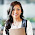High Level Reasoning Coded Inequalities Puzzles Practice Room

Directions (1-5): In these questions, certain symbols have been used to indicate relationships between elements as follows:
A * B means A is either equal to or greater than B.
A \$ B means A is equal to B.
A £ B means A is either equal to or smaller than B.
A & B means A is smaller than B.
A @ B means A is greater than B

In each question, three statements showing relationships have been given, which are followed by two conclusions I & II. Assuming that the given statements are true, find out which conclusion(s) is/are definitely true.
A) if only conclusion I is true.
B) if only conclusion II is true.
C) if either conclusion I or II is true.
D) if neither I nor II is true.
E) if both conclusions I and II are true.

1. Statements: S * K, T & K, K * B
Conclusions: I. S \$ B
II. S @ B

2. Statements: Y \$ Z, H \$ D, Z * D
Conclusions: I. D £ H
II. H £ Z

3. Statements: M @ N, P @ R, P & N
Conclusions: I. P £ M
II. R & N

4. Statements: T & K, K * B, S * K
Conclusions: I. B * T
II. S £ T

5. Statements: P @ R, M @ N, P & N
Conclusions: I. N @ R
II. P & M

Directions (6-10): Read carefully and answer the questions given below:
(i) Six students P, Q, R, S, T and U are in different branches of Engineering, viz. civil, mechanical, chemical, electrical, metallurgy and electronics but not necessarily in the same order.
(ii) Each of them is a resident of a different city viz Mumbai, Calcutta, Chennai, Delhi, Hyderabad and Bangalore. R is the resident of Delhi but he is not in chemical or electrical. T, who is in mechanical, is not the resident of Mumbai or Hyderabad. Q is from Calcutta and he is in electrical. The student from Chennai is in electronics and S is from Mumbai. P is in metallurgy.

6. Which of the following is not the correct combination of student and subject?
a) P-metallurgy
b) Q-electrical
c) U-electronics
d) S-civil
e) All are correct

7. Which student is from Chennai?
a) R
b) U
c) S
d) T
e) None of these

8. P is from which city?
a) Chennai
b) Calcutta
e) None of these

9. Which student is from Bangalore?
a) T
b) Q
c) S
d) T or P
e) None of these

10. R is studying which subject?
a) Electronics
b) Mechanical
c) Metallurgy
e) None of these

1. C
2. B
3. B
4. D
5. E
6. D
7. B
8. C
9. A
10. E

1.Please provide written explanations for Q-1 to Q-5 as explained in session 1 & session 2

1.Sorry for delay reply here is explanation
1. S ≥ K ≥ B
S = B
S > B

2. Y = Z ≥ D = H
D ≤ H
H ≤ Z

3. M > N > P > R
P ≤ M
R < N

4. T < K ≥ B and T < K ≤ S
B ≥ T
S ≤ T

5. M > N > P > R
N > R
P > M

2.5th got typing mistake at P < M

2.explain 5th plz.........

1.Hi Amit,
Already explained in above comment
M > N > P > R
N > R
P < M

3.can you give all the rules for "either or" case in inequality

1.Hi,
I already posted them check below
Coded Inequalities

2.Good explanation.....Thanks

3.you are welcome Priya

4.how to solve inequality when does not equal sign is given